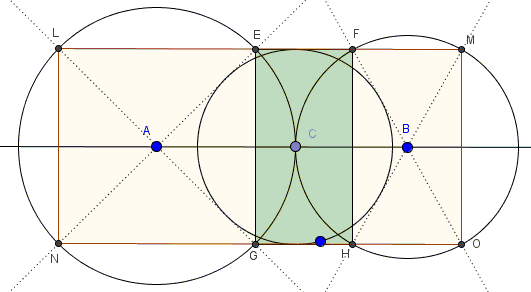# Eye-to-Eye Theorem I

### Problem

$(A)$ and $(B)$ are two circles tangent externally at $C.$ $(C)$ any circle with the radius less than $AC$ and $BC.$ From $A$ and $B$ draw tangents to $(C)$ and mark points $E,G,N,L$ and $F,H,O,M$ where they intersect circles $(A)$ and $(B),$ respectively.Then points $L,E,F,M$ are collinear as are points $N,G,H,O;$ both lines are parallel to $AB,$ making several rectangles, say, $EGHF$ and $LNOM.$

### Hint

There is a good deal of tangent lines and, hence, right triangles. Some of these are similar.

### Solution

I shall prove the assertion only for $EGHF.$

Let $I$ be the foot of the perpendicular from $E$ to $AB;$ $J$ the point of tangency of $AE$ and $(C).$Triangles $AEI$ and $ACJ$ are right and similar because they share an acute angle at $A.$ Their hypotenuses $AC$ and $AE$ are equal as radii of $(A).$ It follows that $\Delta AEI=\Delta ACJ$ and, hence, $EI=CJ$ - the radius of $(C).$

By symmetry, the same holds for $G,F,H,$ making $EGHF$ a rectangle with sides $EF$ and $GH$ tangent to $(C).$

### Acknowledgment

The problem has been posted by Dao Thanh Oai at the CutTheKnotMath facebook page.

The statement admits an easy generalization. I took the liberty of naming the two Eye-to-Eye theorem I and Eye-to-Eye Theorem II.

### Problems with Ophthalmological Connotations

• The Eyeball Theorem
• Eye-to-Eye Theorem II
• The Squinting Eyes Theorem
• Eyeballing a ball
• Praying Eyes Theorem
• Focus on the Eyeball Theorem
• Eyeball Theorem Rectified
• Bespectacled Eyeballs Extension
• Shedding Light on the Ball for Eyeballing
• Eyeballs Projected
• Archimedean Siblings out of Wedlock, i.e., Arbelos
• Rectified, Halved, Sheared, Eyeballs Still Surprise
•